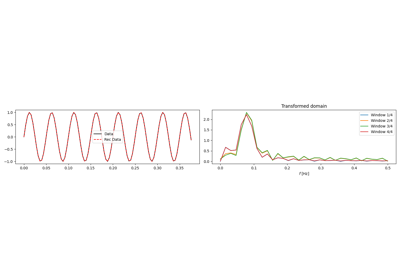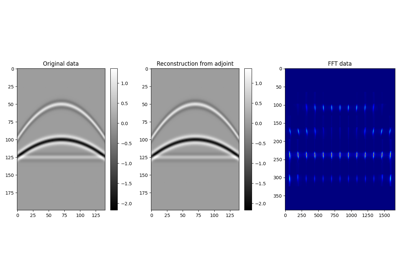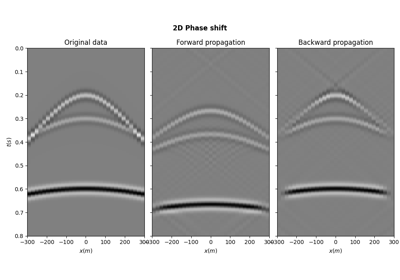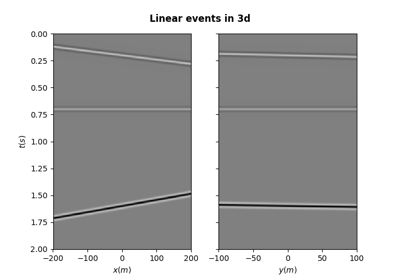# pylops.utils.seismicevents.hyperbolic3d#

pylops.utils.seismicevents.hyperbolic3d(x, y, t, t0, vrms_x, vrms_y, amp, wav)[source]#

Hyperbolic 3D events

Create 3d hyperbolic events given intercept time, root-mean-square velocities, and amplitude of each event

Parameters
xnumpy.ndarray

space axis in x direction

ynumpy.ndarray

space axis in y direction

tnumpy.ndarray

time axis

t0

intercept time at $$x=0$$ of each of hyperbolic event

vrms_x

root-mean-square velocity in x direction for each hyperbolic event

vrms_y

root-mean-square velocity in y direction for each hyperbolic event

amp

amplitude of each hyperbolic event

wavnumpy.ndarray

wavelet to be applied to data

Returns
dnumpy.ndarray

data without wavelet of size $$[n_y \times n_x \times n_t]$$

dwavnumpy.ndarray

data with wavelet of size $$[n_y \times n_x \times n_t]$$

Notes

Each event is created using the following relation:

$t_i(x, y) = \sqrt{t_{0,i}^2 + \frac{x^2}{v_{\text{rms}_x, i}^2} + \frac{y^2}{v_{\text{rms}_y, i}^2}}$

Note that velocities do not have a physical meaning here (compared to the corresponding pylops.utils.seismicevents.hyperbolic2d), they rather simply control the curvature of the hyperboloid along the spatial axes.

## Examples using pylops.utils.seismicevents.hyperbolic3d#1D, 2D and 3D Sliding

1D, 2D and 3D SlidingPatching

PatchingPhaseShift operator

PhaseShift operatorSynthetic seismic

Synthetic seismic